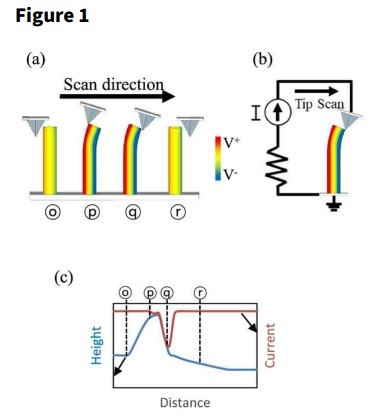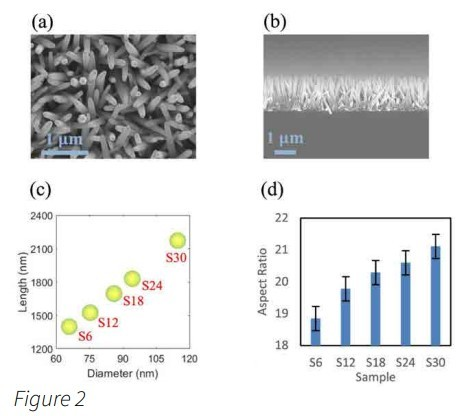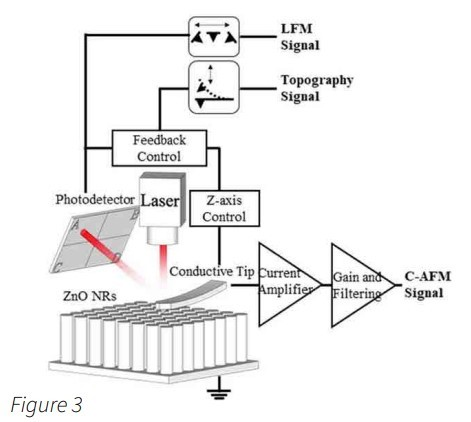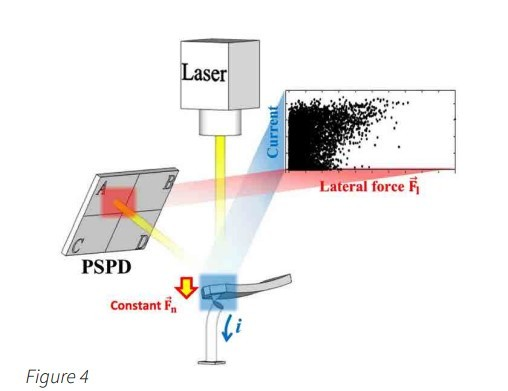# NANOscientific

• Search

### Simultaneous Acquisition Of Current and Lateral Force Signals

Yijun Yang, Kwanlae Kim Department of Manufacturing Systems and Design Engineering (MSDE),

Seoul National University of Science and Technology (SeoulTech),

Seoul, 01811, Republic of Korea Scientific Reports volume 11, Article number: 2904 (2021),

https://www.nature.com/articles/s41598-021-82506-8

### Abstract

Atomic force microscopy (AFM) is central to investigating the piezoelectric potentials of one-dimensional nanomaterials. The AFM probe is used to deflect individual piezoelectric nanorods and to measure the resultant current. However, the torsion data of AFM probes have not been exploited to elucidate the relationship between the applied mechanical force and resultant current. In this study, the effect of the size of ZnO nanorods on the efficiency of conversion of the applied mechanical force into current was investigated by simultaneously acquiring the conductive AFM and lateral force microscopy signals. The conversion efficiency was calculated based on linear regression analysis of the scatter plot of the data. This method is suitable for determining the conversion efficiencies of all types of freestanding piezoelectric nanomaterials grown under different conditions. A pixel-wise comparison of the current and lateral force images elucidated the mechanism of current generation from dense arrays of ZnO nanorods. The current signals generated from the ZnO nanorods by the AFM probe originated from the piezoelectric and triboelectric effects. The current signals contributed by the triboelectric effect were alleviated by using an AFM probe with a smaller spring constant and reducing the normal force.

### Introduction

The piezoelectric effect of ZnO nanorods was first reported by Wang and Song in 20061. Subsequently, an atomic force microscopy (AFM) tip was used to deflect vertically grown ZnO nanorods at the submicron scale. Because ZnO exhibits semiconducting and piezoelectric properties, the strain induced in the individual ZnO nanorods by the AFM tip drives a flow of electric charge carriers through the metal-coated AFM tip and ZnO nanorods. Furthermore, the correlation between the topography signal and conductive atomic force microscopy (C-AFM) signal was analyzed to elucidate the underlying mechanism responsible for the generation of a piezoelectric potential in ZnO nanorods and to detect the current signal via the AFM tip. Through this experimental study, it was shown that the current signal during C-AFM is detected when the AFM tip touches the compressed side of an n-type ZnO nanorod9, as presented in Fig. 1. This comparative analysis of the topography and C-AFM signals also helped elucidate the piezoelectric effect in p-type ZnO nanorods and improved understanding of the effects of the ZnO nanorod growth method used on the piezoelectric power generation characteristics of the resulting nanorods. C-AFM is used preferentially to investigate the piezoelectricity of onedimensional nanomaterials with wurtzite structures, such as CdS, CdSe, ZnS, InN, and GaN.Piezoelectric potential induced by the AFM probe. (a) Generation of piezoelectric potential by deflection of the ZnO nanorod, (b) current passing through the metal-coated AFM tip in contact with the ZnO nanorod, (c) topography and C-AFM signals during the process in (a). The current signals originating from the piezoelectric potential are detected when the AFM tip touches the compressed side of an n-type ZnO nanorod.

Various types of sensors and energy harvesters based on piezoelectric nanomaterials and with a range of structures have been investigated. As these nanomaterials are often subjected to external mechanical forces, understanding their mechanical properties is important. Considering the size of these nanomaterials, lateral force microscopy (LFM) is an effective tool for investigating the elastic moduli of nanomaterials such as ZnO, Au, Si, and W nanowires. To perform mechanical tests accurately on a single nanowire, AFM scanning is conducted along a programmed manipulation path to deflect the nanowire. However, AFM scanning in contact mode also results in the deflection of vertically grown nanorods.

Typically, LFM involves measuring the degree of torsion induced in the AFM cantilever by the surface friction using a position-sensitive photodetector (PSPD). However, when studying the mechanical properties of nanorods, the lateral force applied to the AFM tip may be regarded as the lateral force to which the nanorods are subjected by the AFM tip. Accordingly, by simultaneously monitoring the C-AFM and LFM signals obtained while imaging vertically grown piezoelectric nanorods, the ratio of the output current to the lateral force applied to the ZnO nanorods can be determined. We observed the variations in the C-AFM current signal in response to the application of a lateral force to ZnO nanorods by simultaneously performing C-AFM and LFM. The degree of torsion of an AFM probe can be monitored during AFM operation (including C-AFM) by integrating the AFM instrument with a scanning electron microscope. In particular, Wen suggested that the triboelectric effect and contact potential as well as the piezoelectric effect are responsible for the current signals detected from ZnO nanorods using an AFM probe. However, this method requires a sophisticated experimental arrangement.Conversely, the C-AFM and LFM signals can be readily acquired simultaneously during AFM operation in contact mode. In this work, this novel experimental method was used to study the effect of the ZnO nanorod size on the efficiency of conversion of the mechanical force to which they are subjected into current via the piezoelectric and triboelectric effects. Note that if five ZnO nanorod samples with distinct sizes are deflected by the same AFM tip with a constant normal force, the variation in the lateral force would approximately represent the variation in the net applied mechanical force. Thus, five ZnO nanorod samples with distinct aspect ratios were prepared, and the ratio of the output current to the mechanical force applied to the nanorods was determined. With respect to AFMbased studies of the piezoelectric effect of ZnO nanorods, the choice of the AFM probe may have a determining effect on the results, because the normal force in contact mode is determined by the spring constant of the AFM probe. Therefore, the ratio of the output current to the lateral force was determined using two AFM probes with different spring constants. Finally, we attempted to elucidate the mechanism responsible for the generation of current signals in ZnO nanorods by the AFM tip based on a scatter plot of the current signal versus lateral force and pixel-wise comparison of the C-AFM and LFM images. We confirmed that the piezoelectric and triboelectric effects mainly contribute to the current signals from ZnO nanorods detected by the AFM tip. Furthermore, the contribution of the triboelectric effect is quite large when an AFM probe with a large spring constant is used with a large normal force.

### Results and discussion

#### Vertically grown ZnO nanorods

The lengths and diameters of the vertically grown ZnO nanorods, which were measured with a scanning electron microscopy (SEM) system, are shown in Fig. 2. Overall, the aspect ratio of the ZnO nanorods increases with increasing growth time (see Fig. 2d).Growth of ZnO nanorod samples. (a) Top view and (b) side view of vertically grown ZnO nanorods (S18) and (c) lengths and diameters and (d) aspect ratios of five ZnO nanorod samples (S6‒S30). As the growth time increased, both the length and diameter continuously increased. (a) and (b) were obtained using the scanning electron microscope.

### Representations of current versus lateral force via scatter plot

As stated previously, the C-AFM and LFM signals were acquired simultaneously as an AFM probe scanned the ZnO nanorods. Whereas the LFM signal was transmitted through the PSPD, the C-AFM signal was obtained via the current amplifier (Fig. 3). Thus, there was no cross-talk between these two distinct signals. In this study, all the C-AFM and LFM measurements were obtained over a 10 µm × 5 µm area, which resulted in 512 × 128 data points. The C-AFM and LFM data points were represented as c−afmi, jc-afmi, j and lfmi, j, respectively, using matrix notation. As shown in Fig. 4, a scatter plot was used to represent the correlation between these two sets of data visually. The C-AFM signal from n-type ZnO nanorods has a negative value, whereas the LFM signal during the trace and retrace scans is positive and negative, respectively. Thus, |lc-afmi, jll|c-afmi, j| and ||lfmi, jll|lfmi, j| were used for the computations and graphical representations for simplicity.

Schematic of the experimental setup. Simultaneous acquisition of current and lateral force signals during the scan of vertically grown ZnO nanorods using an AFM probe. The torsional behaviour of the AFM probe was monitored by a laser reflecting from the AFM cantilever into the position-sensitive photodetector. The current signals originating from the ZnO nanorods were detected through the AFM probe and the external circuit with the current amplifier.Scatter plot of current and lateral force signals. During a typical C-AFM measurement, a constant normal force was applied to the ZnO nanorods. The magnitude of the lateral force applied to the nanorods changed continuously. While completing one raster scan, two sets of C-AFM and LFM signals were simultaneously obtained from every data point on the sample surface. The scatter plot composed of these two distinct signals provides useful information for the mechanism of current generation.

### Conclusions

In this study, we performed comprehensive analysis of the piezoelectric and triboelectric effects generated in ZnO nanorods using an AFM probe. By simultaneously acquiring the C-AFM and LFM signals under a constant normal force, the variations in the ratio of the output current to the applied mechanical force with changes in the aspect ratio of the ZnO nanorods could be observed. In particular, two AFM probes with spring constants of 0.2 and 42.0 N/m were used to investigate the effects of the spring constant on the analysis results. The efficiency of the conversion of the lateral force into the output current was computed, and it was found that the efficiency was enhanced with increasing aspect ratio of the ZnO nanorods. In addition, scatter plots of the current versus the lateral force were produced, and the variations in the patterns of the data points with changes in the aspect ratio were analyzed quantitatively using linear regression. The resulting linear model showed that the slope increased with increasing aspect ratio. This finding indicates that there is a transition of the data points in the scatter plots from the lateral force axis (x-axis) towards the current axis (y-axis) as the aspect ratio increases. The variations in the scatter patterns with changes in the aspect ratio of the ZnO nanorods agreed with the results of a pixel-wise comparison of the C-AFM and LFM images. It was confirmed that the current signals were contributed by both the piezoelectric and triboelectric effects in a ZnO nanorod. The effect of triboelectricity on the measured current signals was alleviated by reducing a normal force and the spring constant of an AFM probe. This analysis method is expected to be applicable to all freestanding piezoelectric nanomaterials. Furthermore, it is anticipated to facilitate analysis of the effects of the growth conditions of nanomaterials on the efficiency of conversion of the applied mechanical force into the output current This article has been condensed under Creative Commons Attribution License. The complete article can be found here: https://go.nature.com/2ZGSoeQ.Category